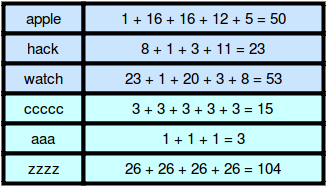# Weighted Uniform Strings HackerRank SolutionWeighted Uniform Strings HackerRank Solution

In this Weighted Uniform Strings HackerRank solution, weighted string is a string of lowercase English letters where each letter has a weight. Character weights are  to  from  to  as shown below:• The weight of a string is the sum of the weights of its characters. For example:• A uniform string consists of a single character repeated zero or more times. For example, ccc and a are uniform strings, but bcb and cd are not.

Given a string, , let  be the set of weights for all possible uniform contiguous substrings of string . There will be  queries to answer where each query consists of a single integer. Create a return array where for each query, the value is Yes if . Otherwise, append No.

## Weighted Uniform Strings HackerRank solution

I will Provide solution in Multiple programming languages for you. If you are not able to find the code in required language then please share in comments so that our team can help you.

### Problem Solution in Python

def weightedUniformStrings(s, queries):
i = 0
count = 1
arr = []
while i < len(s)-1:
if s[i] == s[i+1]:
count += 1
else:
arr.append([ord(s[i])-ord('a')+1, count])
count = 1
i += 1
arr.append([ord(s[i])-ord('a')+1, s.count(s[i])])
ans = []

for q in queries:
flag = False
for i, j in arr:
if q%i == 0 and q <= i*j:
flag = True
break
if flag:
ans.append('Yes')
else:
ans.append('No')
return ans

### Problem Solution in Java

    public static List<String> weightedUniformStrings(String s, List<Integer> queries) {
Set<Integer> weights = new HashSet<>();

for(int i = 0; i < s.length();) {
char c = s.charAt(i);
int weight = c - 'a' + 1;

int j = i+1;
int newWeight = weight;
while(j < s.length() && s.charAt(j) == s.charAt(i)){
newWeight += weight;
j++;
}

i = j;
}

List<String> results = new ArrayList<>();
for (int i = 0; i < queries.size(); i++){
int value = queries.get(i);
if (weights.contains(value)) {
} else {
}
}
return results;
}

### Problem Solution in C++

vector<string> weightedUniformStrings(string s, vector<int> queries) {
vector<string> result;
map<int, int> m;
int count= s-96;
for(int i=1;i<s.size();i++)
{
if(s[i]!=s[i-1])
{
m[count]++;
count=s[i]-96;
}
else
{
m[count]++;
count+=s[i]-96;
}
}
m[count]++;

for(int i=0;i<queries.size();i++)
{
if(m[queries[i]]==0)
result.push_back("No");
else
result.push_back("Yes");
}
return result;
}

### Problem Solution in JavaScript

function weightedUniformStrings(s, queries) {
const weights = new Set();
const calculateWeight = s => s.length * (s.charCodeAt() - 'a'.charCodeAt() + 1);
let left = 0;

for(let right = 0; right < s.length; right++) {
if(s[left] !== s[right]) {
left = right;
}
const ss = s.substring(left, right + 1);
}